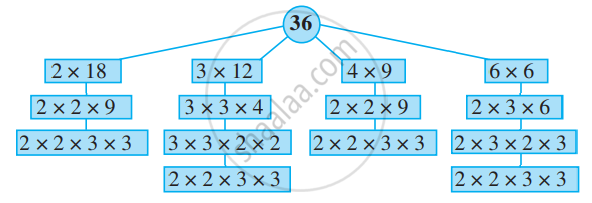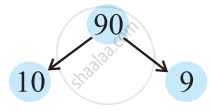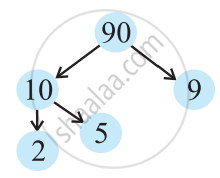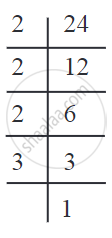# Prime Factorisation:

## a) Prime Factorisation:

When a number is expressed as a product of its factors we say that the number has been factorised. Thus, when we write 56 = 8 × 7, we say that 56 has been factorised. This is one of the factorisations of 56. The others are:

 56 = 2 × 28 = 2 × 2 × 14 = 2 × 2 × 2 × 7 56 = 4 × 14 = 2 × 2 × 14 = 2 × 2 × 2 × 7 56 = 7 × 8 = 7 × 2 × 4 = 7 × 2 × 2 × 2 56 = 8 × 7 = 2 × 4 × 7 = 2 × 2 × 2 × 7

In all the above factorisations of 56, we ultimately arrive at only one factorisation 2 × 2 × 2 × 7. In this factorisation the only factors 2 is a prime number. Such a factorisation of a number is called prime factorisation.

Let us check this for the number 36.The prime factorisation of 36 is 2 × 2 × 3 × 3. i.e. the only prime factorisation of 36.

## Factor Tree:

Consider the number 90.

Think of a factor pair say, 90 = 10 × 9.Now think of a factor pair of 10, 10 = 2 × 5.Write factor pair of 99 = 3 × 3

## b) Factorising a Number into its Prime Factors:

A simple but important rule given by Euclid is often used to find the GCD or HCF and LCM of numbers. The rule says that any composite number can be written as the product of prime numbers.

Let us learn how to find the prime factors of a number.

Example: Write the number 24 in the form of the product of its prime factors.

Method for finding prime factors

### i) Vertical arrangement:24 = 2 × 2 × 2 × 3

ii) Horizontal arrangement:

24 = 2 × 12
= 2 × 2 × 6...12 is factorised
= 2 × 2 × 2 × 3...6 is factorised.
The prime factors are 2 and 3.

Remember: To write a given number as a product of its prime factors is to factorise it into primes.

#### Example

Factorise into primes: 117.

Vertical arrangement:

 3 117 3 39 13 13 1

117 = 3 × 3 × 13.

Horizontal arrangement:

117 = 13 × 9
117 = 13 × 3 × 3
117 = 3 × 3 × 13.

#### Example

Factorise into primes: 250.

Vertical arrangement:

 2 250 5 125 5 25 5 5 1

250 = 2 × 5 × 5 × 5.

Horizontal arrangement:

250 = 2 × 125
250 = 2 × 5 × 25
250 = 2 × 5 × 5 × 5.

#### Example

Find the prime factors of 40.

Vertical arrangement:

 2 40 2 20 2 10 5 5 1

40 = 2 × 2 × 2 × 5

Horizontal arrangement:

40 = 10 × 4
40 = 5 × 2 × 2 × 2.

If you would like to contribute notes or other learning material, please submit them using the button below.

### Shaalaa.com

Prime Factorisation - Factor Trees Method [00:11:41]
S
0%